# ISEE Lower Level Math : How to find the missing part of a list

## Example Questions

← Previous 1 3

### Example Question #1 : How To Find The Missing Part Of A List

What number comes next in this sequence?

2, 3, 5, 7, 11, 13, __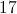Explanation:

Looking at this sequence, the most obvious thing may be the string of odd numbers. But as you look more closely, you'll notice that the 9 is missing and that there is a 2 in the list. Whenever you see a 2 in a list of odd numbers, your "prime number sensor" (not a real thing) should be on alert. As this is a list of consecutive prime numbers, the next number in the sequence is 17.

### Example Question #2 : How To Find The Missing Part Of A List

Define two sets as follows: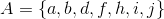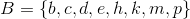How many elements are in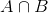?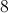Explanation:is the intersection of setsand- the set of all elements that appear in both sets. As can be seen, those elements are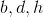- making 3 the correct choice.

### Example Question #3 : How To Find The Missing Part Of A List

Use the number sequence to answer the question.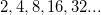What is the next number in the sequence?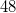Explanation:

The numbers in this sequence follow a pattern; each new number is twice the one that came before it. To find the next number in the sequence, multiply by two.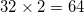.

### Example Question #4 : How To Find The Missing Part Of A List

Find the missing number in the sequence: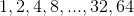16

20

24

12

16

Explanation:

The pattern of the sequence is to multiply each number byto get to the next number.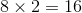and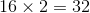### Example Question #5 : How To Find The Missing Part Of A List

What is the next number in this sequence?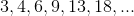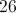Explanation:

Notice that the numbers in this list are growing at an increasing rate.

First you add: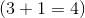Then you add: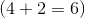Then you add: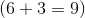.

By the time you get to the 18, it is time to add 6: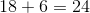### Example Question #6 : How To Find The Missing Part Of A List

What number comes next in the sequence?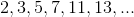Explanation:

The sequence of numbers represents prime numbers, beginning with two. The next prime number in the list would be.

Note thatis not a prime number, since it is a multiple ofand.

### Example Question #7 : How To Find The Missing Part Of A List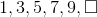What is the next number in this series?Explanation:

Since the pattern is adding 2, you must add 2 to 9 to get 11.

### Example Question #8 : How To Find The Missing Part Of A List

What is the missing number in this sequence?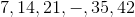Explanation:

In this sequence, each number is a multiple of, and each number increases incrementally by. The multiple ofthat is betweenandis; therefore,is the correct answer.

### Example Question #9 : How To Find The Missing Part Of A List

What is the next number in the sequence?Explanation:In the sequence, each subsequent number is larger than the previous number by 6.

To calculate the next number, 6 should be added to 24, resulting in 30. Thus, 30 is the correct answer.

### Example Question #10 : How To Find The Missing Part Of A List

What is the value ofin this sequence?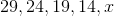None of theseExplanation:

In this sequence, each subsequent number is 5 less than the preceding number. As a result, thewill be equal to 9, because 9 is 5 less than 14, which is the preceding number.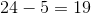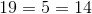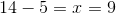← Previous 1 3

### All ISEE Lower Level Math Resources